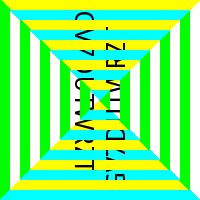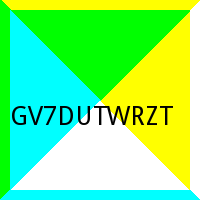# Pwnium CTF - ROT Writeup

I wanted to do a writeup on SOMETHING from this CTF. So I picked the task I spent the most time on: ROT, a programming challenge worth 300 points.

The challenge said "nc 41.231.53.40 9090" and "ROT 90, ROT -90, ROT 90..." so as an obvious first step I connected to the server to see what I had to do.

``````nc 41.231.53.40 9090
``````

About fifty lines of base64 encoded data and then an answer prompt. Okay so decode, solve for the flag, and submit it. No, not that simple! The connection to the server would close after about 3 seconds and each time that I connected the challenge base64 data changed. Whatever I had to program needed to work fast and provide the answer back. Since sockets are nice and simple in Python, it's what I chose to write this in. Alright, now time to figure out what that base64 is...

``````#!/usr/bin/env python
import socket
import base64
s = socket.socket(socket.AF\_INET, socket.SOCK\_STREAM)
s.connect(("41.231.53.40", 9090))
print(base64.b64decode(s.recv(30000)))
``````

Looks like a file and checking the header confirms: it's a PNG... with the "Answer: " prompt still tacked onto the end. Cool so split the output by newline, write it to a file, and check it out:The whole ROT idea is starting to take shape. It looks like the answer is a string of characters but mutated somehow. According to the hint 90°, -90°, 90°, -90°, etc.

I decided to start from the outside and go in. The outermost strip does not have a character on it so I ignore it in this solution and start at the next box in. The 2nd section needed to be rotated 90° so I used Pillow (a maintained fork of the Python Image Library (PIL)) to manipulate the image. The image is 200px by 200px and has 10 boxes including the outermost one.

Pillow allows selection of a square, rotation, and pasting back onto the original image; just what I needed. The idea is to select a box rotate it 90°, make the box one section smaller, and then rotate the rest back -90° and then repeat for the next section with -90° and 90° back. Since there are 10 sections over a 200x200 image, each side of a box is 10px. PIL box selections can be done by specifying the x and y coordinates of the upper left and the lower right corners in a tuple. Starting at ( 10, 10, 190, 190 ) and increasing the left bounds by 10 each and decreasing the right bounds by 10 while rotating until the middle is reached results in the solved image.``````def rotateImg():
img = Image.open(sys.argv)
left, upper, right, lower = (10, 10, 190, 190)
for i in range(0, 9):
box = ( left, upper, right, lower )
region = img.crop(box)
if (i % 2) == 0:
region = region.transpose(Image.ROTATE_90)
else:
region = region.transpose(Image.ROTATE_270)
img.paste(region, box)
left += 10
upper += 10
right -= 10
lower -= 10
box = ( left, upper, right, lower )
region = img.crop(box)
if (i % 2) == 0:
region = region.transpose(Image.ROTATE_270)
else:
region = region.transpose(Image.ROTATE_90)
img.paste(region, box)
img.save("ans.png", "PNG")
``````

Now the issue of recognizing the text and sending the answer. The readbot library uses the tesseract-ocr engine to recognize text. Now all that needed to be done was implement it and send back the answer. Sure enough, after a couple of incorrect reads the flag was returned!

``````./prog300.py lol.png
Tesseract Open Source OCR Engine v3.03 with Leptonica
GV7DUTWRZT
<strong>Flag: Pwnium{b1a371c90da6a1d2deba2f6ebcfe3fc0}</strong>
``````

Here's the final code:

``````#!/usr/bin/env python
import base64
import socket
import sys
from PIL import Image
def getImage():
PORT = 9090
s = socket.socket(socket.AF_INET, socket.SOCK_STREAM)
s.connect(("41.231.53.40", PORT))
with open(sys.argv, "w") as file:
file.write(base64.b64decode(s.recv(30000).split('\n')))
return s
def rotateImg():
img = Image.open(sys.argv)
left, upper, right, lower = (10, 10, 190, 190)
for i in range(0, 9):
box = ( left, upper, right, lower )
region = img.crop(box)
if (i % 2) == 0:
region = region.transpose(Image.ROTATE_90)
else:
region = region.transpose(Image.ROTATE_270)
img.paste(region, box)
left += 10
upper += 10
right -= 10
lower -= 10
box = ( left, upper, right, lower )
region = img.crop(box)
if (i % 2) == 0:
region = region.transpose(Image.ROTATE_270)
else:
region = region.transpose(Image.ROTATE_90)
img.paste(region, box)
img.save("ans.png", "PNG")
def ocrthatbitch(img):